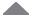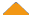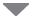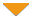Run Code  | API  | Code Wall  | Misc  | Feedback  | Login  | Theme  | Privacy  | Patreon

## Query Anisa YP

 run  | edit  | history  | help0# SOAL 1

`SELECT * FROM [shipping] WHERE ADDRESS_CITY = 'JABODETABEK'`

# SOAL 2

```SELECT A.*, B.jumlah_order FROM [member] A LEFT JOIN (SELECT member_id, COUNT(1) as jumlah_order FROM [order] GROUP BY member_id) B ON A.member_id = b.member_id ```

# SOAL 3

```SELECT address_city, sum(jumlah_order) as jumlah_order FROM ( SELECT A.*, B.jumlah_order FROM [member] A LEFT JOIN (SELECT member_id, COUNT(1) as jumlah_order FROM [order] GROUP BY member_id) B ON A.member_id = b.member_id ) A GROUP BY address_city ```

# SOAL 4

```SELECT address_city, sum(jumlah_order) as jumlah_order FROM ( SELECT A.*, B.jumlah_order FROM [member] A LEFT JOIN (SELECT member_id, COUNT(1) as jumlah_order FROM [order] GROUP BY member_id) B ON A.member_id = b.member_id ) A GROUP BY address_city ORDER BY jumlah_order DESC ```

# SOAL 5

```SELECT A.*, C.address_city as Shipping_address_city FROM [member] A LEFT JOIN [order] B ON A.member_id = B.member_id LEFT JOIN [shipping] C ON B.order_id = C.order_id WHERE A.address_city <> C.address_city ```

# SOAL 6

```SELECT A.*, B.jumlah_belanja, B.total_order, (B.total_order/B.jumlah_belanja) as rata2_belanja FROM [member] A LEFT JOIN (SELECT member_id, COUNT(1) as jumlah_belanja, SUM(total) as total_order FROM [order] GROUP BY member_id) B ON A.member_id = B.member_id WHERE jumlah_belanja >= 2 ```

# SOAL 7

```SELECT * FROM [member] WHERE member_id IN ( SELECT member_id FROM [order] WHERE MONTH(CREATED) IN ( 1 , 3 ) AND member_id NOT IN ( SELECT member_id FROM [order] WHERE MONTH(CREATED) = 2 ) GROUP BY member_id ) ```

by  Kuncoro Wicaksono, 4 years ago